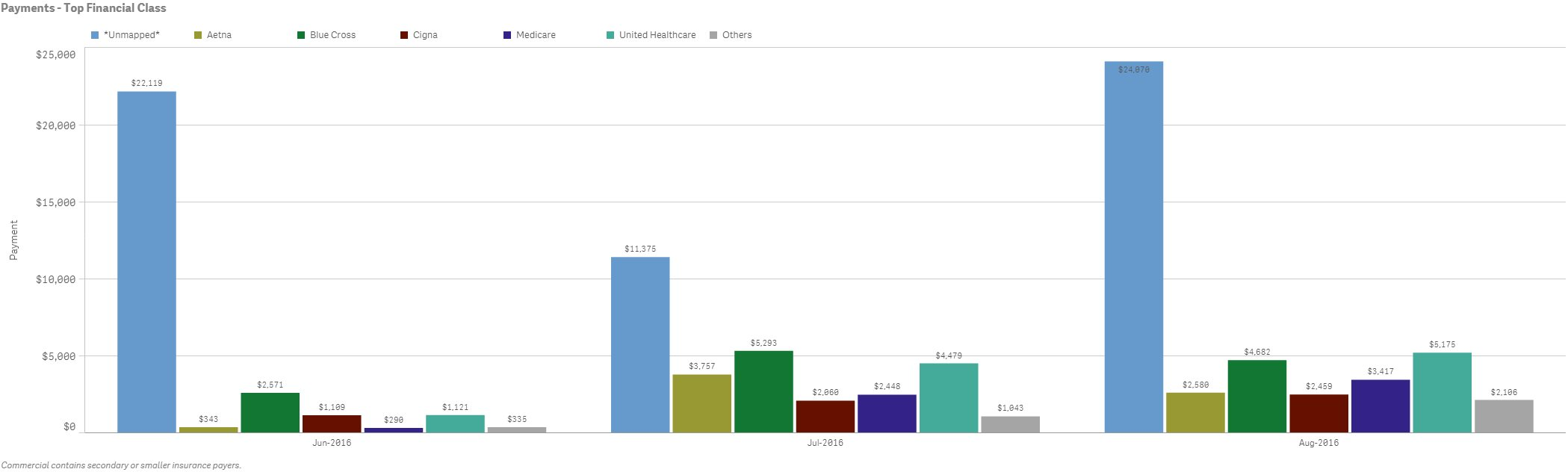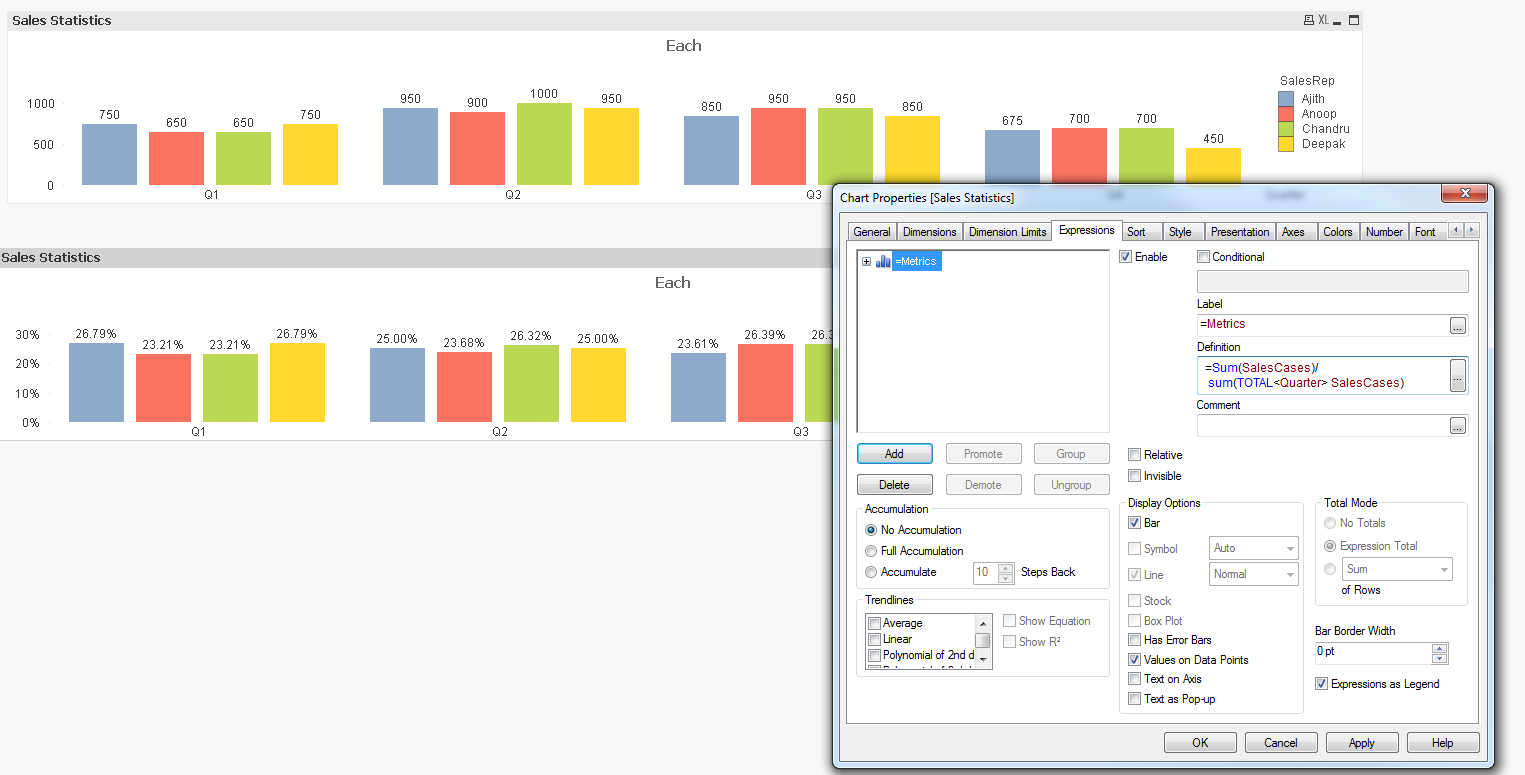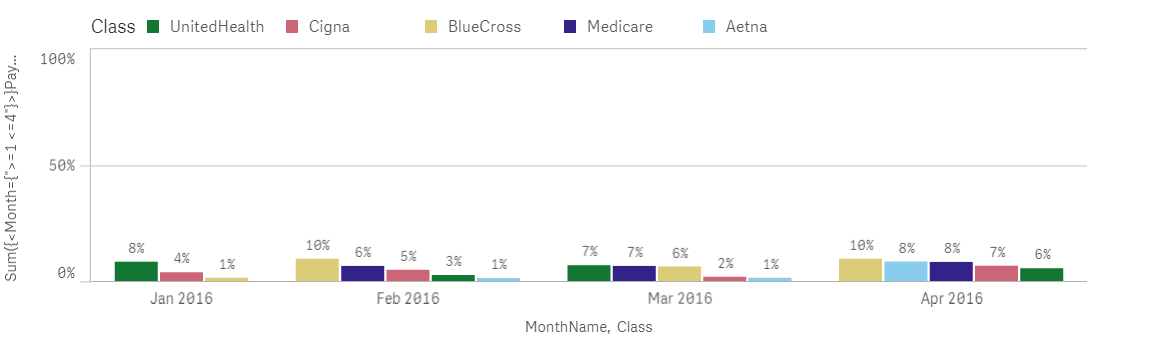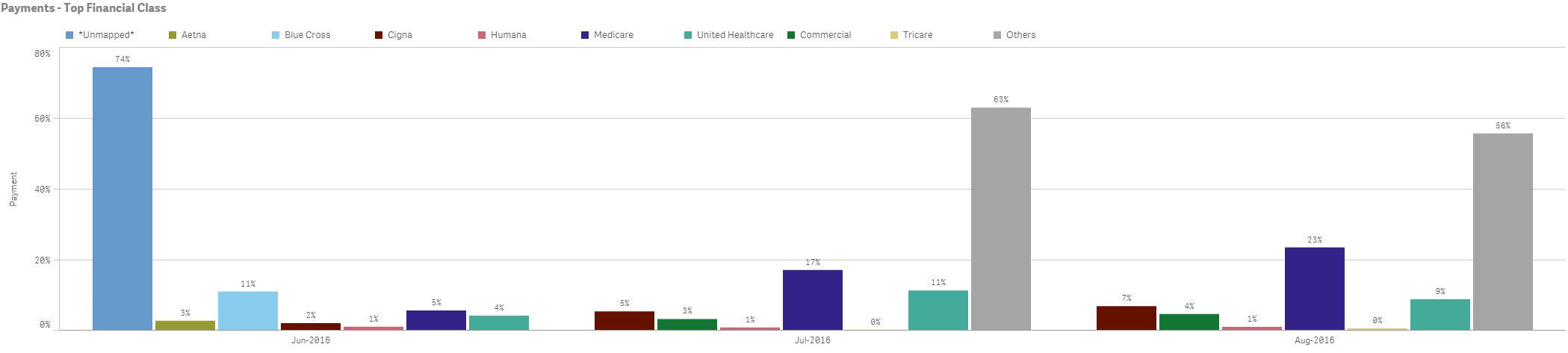# New to Qlik Sense

Discussion board where members can get started with Qlik Sense.

Announcements
Qlik® Product Spotlight: Discover what’s possible. Get more from our products.
See for yourself. Register today.Not applicable

## % by month

Hello:

Dimension: MonthYear and then FinancialClass

Measure: -Sum({<[TransPostedDateCalendar.MonthsAgo]={">=1<=4"}>}Payment_Total)

How do I calculate % by month? E.g. For June 2016, total payments was \$27,888. The financial class *Unmapped* would be 79%, Blue Cross would be 9%, etc. I need each month to total 100%.

Thank you.Tags (1)
1 Solution

Accepted Solutions
Not applicable

## Re: % by month

Try

(Sum({<[TransPostedDateCalendar.MonthsAgo]={">=1<=4"}>}Payment_Total))

/

(Sum(Total <Month>{<[TransPostedDateCalendar.MonthsAgo]={">=1<=4"}>}Payment_Total))

9 Replies
Not applicable

## Re: % by month

Hi,

Maybe try something like this:

Sum({<[TransPostedDateCalendar.MonthsAgo]={">=1<=4"}>}Payment_Total) / Aggr(Sum({<[TransPostedDateCalendar.MonthsAgo]={">=1<=4"}>}Payment_Total), MonthYear)

Hope it helps

Not applicable

## Re: % by month

Try

(Sum({<[TransPostedDateCalendar.MonthsAgo]={">=1<=4"}>}Payment_Total))

/

(Sum(Total <Month>{<[TransPostedDateCalendar.MonthsAgo]={">=1<=4"}>}Payment_Total))Not applicable

## Re: % by month

Thanks Sinan. However, this didn't work. I need each of the FinancialClass to show a % and then all the FinancialClass within a month to total 100%.Not applicable

## Re: % by month

Thanks Neelam. However, your suggestion didn't work. It gave me an error and didn't display anything.

Not applicable

## Re: % by month

Can you share some sample data

Not applicable

## Re: % by month

Check this out..Its works for me...Not applicable

## Re: % by month

Try this:

Sum({<[TransPostedDateCalendar.MonthsAgo]={">=1 <=4"}>}Payment_Total)/

Sum(TOTAL {<[TransPostedDateCalendar.MonthsAgo]={">=1 <=4"}>}Payment_Total)

To get 100% for each month add the Monthname to the expression;

Sum({<[TransPostedDateCalendar.MonthsAgo]={">=1 <=4"}>}Payment_Total)/

Sum(TOTAL<MonthName>{<[TransPostedDateCalendar.MonthsAgo]={">=1 <=4"}>}Payment_Total)

PS: Number format is set to simple --> 12%

See attached example:Not applicable

## Re: % by month

Do you have two dimension and 1 expression or do you have one dimension and 7 expressions in your chart?

If you have 2 dimensions and 1 expression, then something like this should work

Sum(Measure)/Sum(TOTAL <Dimension1> Measure)

If you have 1 dimension and 7 expressions, then something like this should work

Expression1/RangeSum(Expression1, Expression2, Expression3, Expression4, Expression5, Expression6, Expression7)Not applicable

## Re: % by month

It worked neelam! Thank you so much!Community Browser